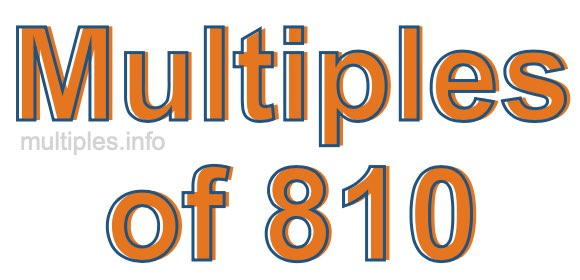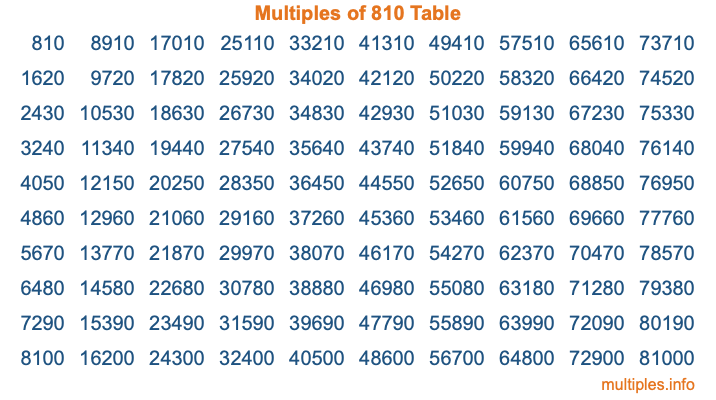Multiples of 810Welcome to the Multiples of 810 page. Here we will first teach you everything you will ever need to know about the multiples of 810, and then give you a study guide summary of everything we taught you to make sure you remember it all. Use this page to look up facts and learn information about the multiples of 810. This page will make you a multiples of eight hundred ten expert!

Definition of Multiples of 810
Multiples of 810 are all the numbers that when divided by 810 equal an integer. Each of the multiples of 810 are called a multiple. A multiple of 810 is created by multiplying 810 by an integer.

Therefore, to create a list of multiples of 810, you start with 1 multiplied by 810, then 2 multiplied by 810, then 3 multiplied by 810, and so on for as long as you want. Thus, the list of the first five multiples of 810 is 810, 1620, 2430, 3240, and 4050. To see a larger list of multiples of 810, see the printable image of Multiples of 810 further down on this page. We also have a category where you can choose any nth multiple of 810.

Multiples of 810 Checker
The Multiples of 810 Checker below checks to see if any number of your choice is a multiple of 810. In other words, it checks to see if there is any number (integer) that when multiplied by 810 will equal your number. To do that, we divide your number by 810. If the the quotient is an integer, then your number is a multiple of 810.

Is  a multiple of 810?

Least Common Multiple of 810 and ...
A Least Common Multiple (LCM) is the lowest multiple that two or more numbers have in common. This is also called the smallest common multiple or lowest common multiple and is useful to know when you are adding our subtracting fractions. Enter one or more numbers below (810 is already entered) to find the LCM.

Check out our LCM Calculator if you need more details about the Least Common Multiple or if you need the LCM for different numbers for adding and subtraction fractions.

nth Multiple of 810
As we stated above, 810 is the first multiple of 810, 1620 is the second multiple of 810, 2430 is the third multiple of 810, and so on. Enter a number below to find the nth multiple of 810.

th multiple of 810

Multiples of 810 vs Factors of 810
810 is a multiple of 810 and a factor of 810, but that is where the similarities end. All postive multiples of 810 are 810 or greater than 810. All positive factors of 810 are 810 or less than 810.

Below is the beginning list of multiples of 810 and the factors of 810 so you can compare:

Multiples of 810: 810, 1620, 2430, 3240, 4050, etc.

Factors of 810: 1, 2, 3, 5, 6, 9, 10, 15, 18, 27, 30, 45, 54, 81, 90, 135, 162, 270, 405, 810

As you can see, the multiples of 810 are all the numbers that you can divide by 810 to get a whole number. The factors of 810, on the other hand, are all the whole numbers that you can multiply by another whole number to get 810.

It's also interesting to note that if a number (x) is a factor of 810, then 810 will also be a multiple of that number (x).

Multiples of 810 vs Divisors of 810
The divisors of 810 are all the integers that 810 can be divided by evenly. Below is a list of the divisors of 810.

Divisors of 810: 1, 2, 3, 5, 6, 9, 10, 15, 18, 27, 30, 45, 54, 81, 90, 135, 162, 270, 405, 810

The interesting thing to note here is that if you take any multiple of 810 and divide it by a divisor of 810, you will see that the quotient is an integer.

Multiples of 810 Table
Below is an image of the first 100 multiples of 810 in a table. The table is in chronological order, column by column. The first column has the first ten multiples of 810, the second column has the next ten multiples of 810, and so on.The Multiples of 810 Table is also referred to as the 810 Times Table or Times Table of 810. You are welcome to print out our table for your studies.

Negative Multiples of 810
Although not often discussed or needed in math, it is worth mentioning that you can make a list of negative multiples of 810 by multiplying 810 by -1, then by -2, then by -3, and so on, to get the following list of negative multiples of 810:

-810, -1620, -2430, -3240, -4050, etc.

Multiples of 810 Summary
Below is a summary of important Multiples of 810 facts that we have discussed on this page. To retain the knowledge on this page, we recommend that you read through the summary and explain to yourself or a study partner why they hold true.

There are an infinite number of multiples of 810.

A multiple of 810 divided by 810 will equal a whole number.

810 divided by a factor of 810 equals a divisor of 810.

The nth multiple of 810 is n times 810.

The largest factor of 810 is equal to the first positive multiple of 810.

810 is a multiple of every factor of 810.

810 is a multiple of 810.

A multiple of 810 divided by a divisor of 810 equals an integer.

810 divided by a divisor of 810 equals a factor of 810.

Any integer times 810 will equal a multiple of 810.

Multiples of a Number
Here you can get the multiples of another number, all with the same attention to detail as we did for multiples of 810 on this page.

Multiples of
Multiples of 811
Did you find our page about multiples of eight hundred ten educational? Do you want more knowledge? Check out the multiples of the next number on our list!